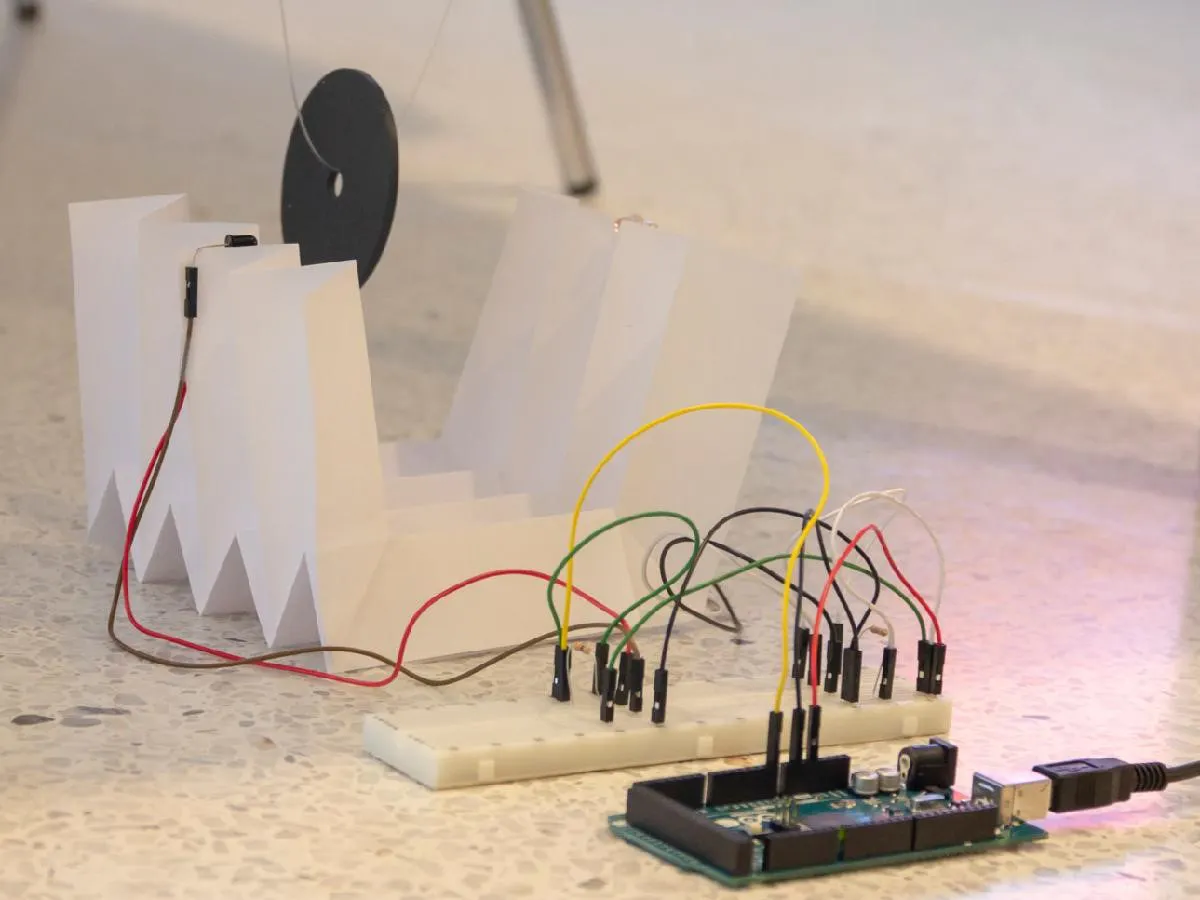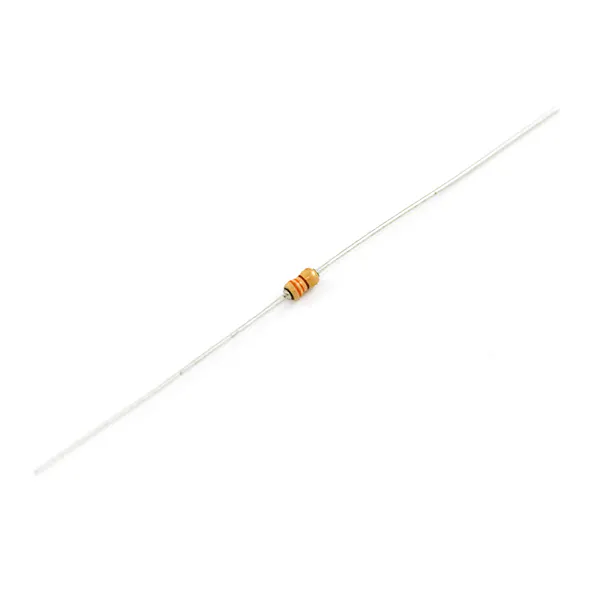Project tutorial# Arduino Pendulum Physics Lab © MIT

Easy to build pendulum to get data from an Arduino, analyze it to find the period, and connect theory with experiment.

• 4,732 views
• 15 respects

## Components and supplies

 Arduino Mega 2560 R3
×1
 SparkFun USB Cable A to B - 6 Foot
×1
 SparkFun Infrared Emitters and Detectors
×1Resistor 330 ohm
×1
 SparkFun Resistor 10K Ohm 1/4 Watt PTH
×1
×1
 SparkFun Jumper Wires Premium 6" M/M
×1
 SparkFun Jumper Wires Premium 12" M/F
×1
 Fender Washer
×1
 String or fishing line
×1
×1
 Sheets of paper
×1

## Apps and online services

### How to Build the Photo-Gate

This video shows how to make the photo-gate origami structure.

Refer to the schematics section for building the circuit.

### How to Run This Project in MATLAB

You can download this project from the File Exchange and run it directly in MATLAB.

### Acquiring and Analyzing Data

Connect MATLAB® to the Arduino®

``````a = arduino()
``````

If you see an error message, you may have already connected MATLAB to an Arduino by running the code more than once. You can simply ignore the error message and continue.

Specify the data pin on the Arduino (yellow wire in the schematic).

``````photogatePin = 'A0';
``````

Define the number of samples to read.

``````numSamples = 2^10;
``````

Initialize an empty vector for collecting voltage data.

``````v = zeros(1,numSamples)
``````

Set the pendulum swinging and read the voltage in a loop.

Time how long it takes to read `numSamples` with `tic` and `toc`, which returns results in units of seconds.

``````tic
for s = 1 : numSamples
end
clockTime = toc
``````

If you do not have an Arduino, but would like to follow along with the rest of this project, load the set of example data by uncommenting and running the following code:

``````% load data.mat;
``````

The time vector is

``````t = linspace(0,clockTime,numSamples);
``````

Plot the voltage time signal.

``````plot(t,v);
xlabel 'time (s)';
ylabel 'voltage (V)';
``````

To find the period of the pendulum from the voltage signal, find the peak to peak time differences.

First find the high points by thresholding the voltage by defining a logical vector

``````isHigh = v > mean(v);
``````

then use the vector to logically index into `t` and `v`.

``````tHigh = t(isHigh);
vHigh = v(isHigh);
``````

Plot the results.

``````plot(t,v,'.-',tHigh,vHigh,'o');
xlabel('time (s)');
ylabel('voltage (V)');
``````

Compute the time between high point samples and display the set's histogram. If the pendulum swing is slow enough, you may have multiple samples in a row that read as high points. Zoom into the voltage signal plot above to see this.

``````dt = diff(tHigh);
histogram(dt);
xlabel('dt');
ylabel('count');
``````

The first peak in the histogram is made up of samples within a single peak. The other bins are made of samples across peaks. Threshold the `dt` samples based on your observation of the histogram to identify the samples across peaks.

``````dtThreshold = 0.3;
``````

The logical vector that picks out the elements of `dt` that form the gaps across peaks is

``````isGap = dt > dtThreshold;
``````

The elements of `tHigh `that are on the falling edges of the peaks are

``````isEdge = [isGap false];
``````

where we appended false to `isGap` to account for the last peak, which has no peak after it to form a gap (or valley). The `t`, `v` values of the falling edges of the peaks are therefore

``````tEdge  = tHigh(isEdge);
vEdge  = vHigh(isEdge);
plot(t,v,'.-',tEdge,vEdge,'o');
``````

The time differences between the falling edges of the peaks are

``````dtEdge = diff(tEdge);
``````

Check a histogram to see if it is physically valid to talk about a mean peak-to-peak time, i.e. if it is gaussian.

``````histogram(dtEdge);
xlabel 'peak-to-peak time (s)';
ylabel 'count';
``````

The period of the pendulum is the time it takes to complete a full swing, which includes

• breaking the photo-gate going in one direction
• breaking it going in reverse
• breaking it going in the original direction,

to form a total of 3 peaks. So, the period is twice the measured mean peak-to-peak time.

``````T = 2 * mean(dtEdge)
``````

The pendulum's period and length are related to the acceleration due to gravity by

so knowing the pendulum's period and length,

``````L = 0.62; % units of meters
``````

we can find the acceleration due to gravity according to

``````g_value = (2*pi/T)^2 * L % m s^-2
``````

## Code

##### Arduino Pendulum Physics LabMATLAB
https://www.mathworks.com/matlabcentral/fileexchange/68392-arduino-pendulum-physics-lab
```No preview (download only).
```

## Schematics#### Ultrasonic Map-Maker using an Arduino Yun

Project tutorial by Satyavrat Wagle

• 51,927 views
• 152 respects

#### Test DHT22 Sensors with Arduino and MATLAB

Project tutorial by dancili

• 4,460 views
• 20 respects

#### Measure and Analyze Tide Levels with ThingSpeak and MATLAB

Project tutorial by Team MATLAB IoT

• 10,149 views
• 27 respects

#### Make it Possible with Physics - Ultrasonic Thermometer!

Project showcase by berkeralpz

• 6,198 views
• 13 respects

#### Drive with PID Control on an Arduino Mega 2560

Project tutorial by Team MATLAB Makers

• 12,709 views